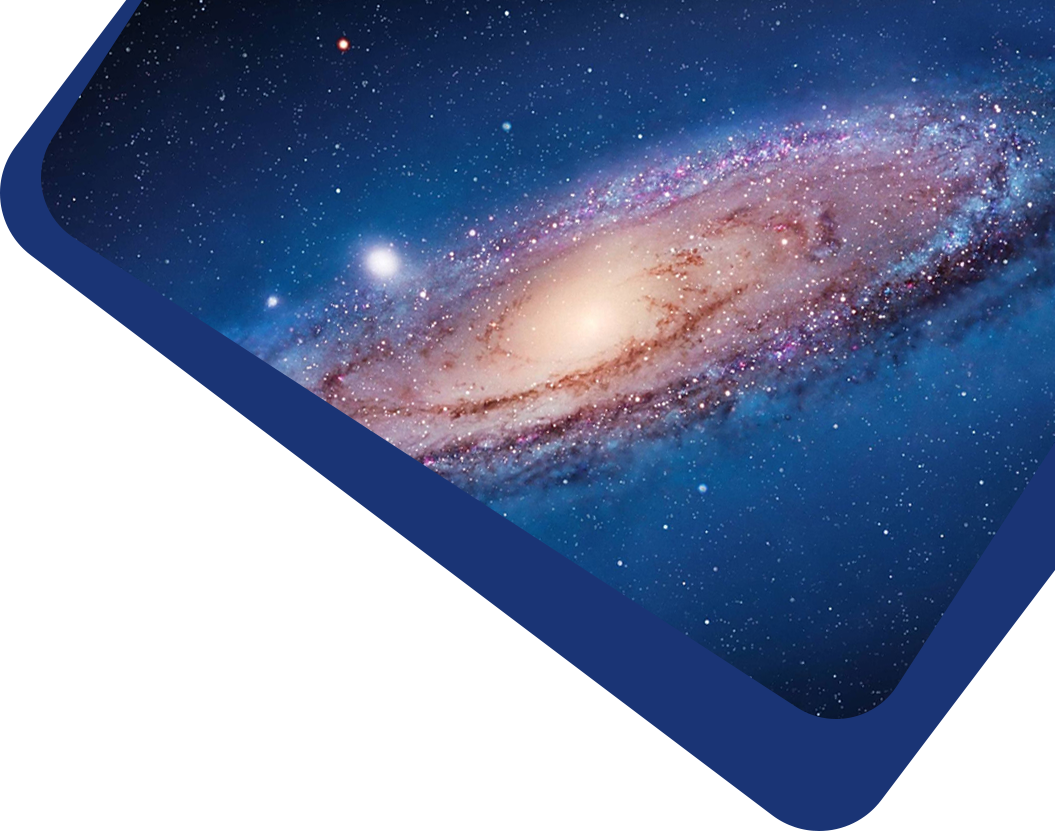Vol 23, No 1

## Modeling the Vertical Distribution of the Milky Way’s Flat Subsystem Objects

#### Igor’ I. Nikiforov, Vadim A. Usik and Angelina V. Veselova

Abstract

This paper is an initial stage of consideration of the general problem of joint modeling of the vertical structure of a Galactic flat subsystem and the average surface of the disk of the Galaxy, taking into account the natural and measurement dispersions. We approximate the average surface of the Galactic disk in the region covered by the data with a general (polynomial) model and determine its parameters by minimizing the squared deviations of objects along the normal to the model surface. The smoothness of the model, i.e., its order n, is optimized. An outlier elimination algorithm is applied. The developed method allows us to simultaneously identify significant details of the Galactic warping and estimate the offset z of the Sun relative to the average (in general, non-flat) surface of the Galactic disk and the vertical scale of the object system under consideration for an arbitrary area of the disk covered by data. The method is applied to data on classical Cepheids. Significant local extremes of the average disk surface model were found based on Cepheid data: the minimum in the first Galactic quadrant and the maximum in the second. A well-known warp (lowering of the disk surface) in the third quadrant has been confirmed. The optimal order of the model describing all these warping details was found to be no = 4. The local (for a small neighborhood of the Sun, no = 0) estimate of $$z_{\odot}=28.1\pm\left.6.1\right|_{\mathrm{stat}\left.\pm1.3\right|_{mathrm{cal}}$$ pc is close to the non-local (taking into account warping, no = 4) $$z_{\odot}=27.1\pm\left.8.8\right|_{\mathrm{stat}\left._{-1.2}^{+1.3}\right|_{mathrm{cal}}$$ pc (statistical and calibration uncertainties are indicated), which suggests that the proposed modeling method eliminates the influence of warping on the z estimate. However, the non-local estimate of the vertical standard deviation of Cepheids $$\sigma_{\rho}=132.0\pm\left.3.7\right|_{\mathrm{stat}}\left._{-5.9}^{+6.3}\right|_{mathrm{cal}}$$ pc differs significantly from the local $$\sigma_{\rho}=76.5m\left.4.4\right|_{\mathrm{stat}}\left._{-3.4}^{+3.6}\right|_{mathrm{cal}}$$ pc, which implies the need to introduce more complex models for the vertical distribution outside the Sun’s vicinity.

Keywords

Full Text
Refbacks